# Multiplying Fractions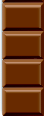Example 1: Tyrone had a chocolate bar, of which he gave one-half to his friend Nora. Nora gave one-half of her piece to her friend Eduardo. What fractional part of the original chocolate bar did Eduardo get?

Analysis: We are taking part of a part. So we must end up with an even smaller part. The word OF means "times", which indicates that we need to multiply.

Solution: One-half of one whole is one-half. One-half of one-half is one-fourth.

Step 1: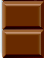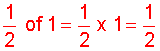Step 2: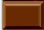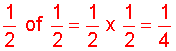The solution above should make sense given our knowledge of fractions. We also can check this solution using our knowledge of decimals as follows: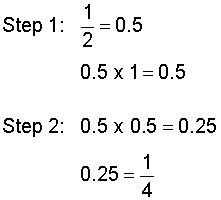Let's look at another example.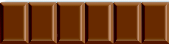Example 2: Estelle had a chocolate bar, of which she gave one-half to her friend Lourdes. Lourdes gave two-thirds of her piece to her friend Donna. What fractional part of the original chocolate bar did Donna get?

Analysis: The word OF means "times". We will multiply one-half by two-thirds.

Solution: We can show the solution in three steps.

Step 1:Step 2: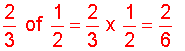Step 3: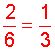Answer: Donna got one-third of the original chocolate bar.

The original chocolate bar consisted of 6 sections. Estelle gave Lourdes one-half of those, which is 3 sections. Lourdes gave Donna two-thirds of those, which is 2 sections. As you can see, 2 sections is one-third of 6. This leads us to the following procedure for multiplying fractions.

Procedure: To multiply two fractions:

1. Multiply the numerators by the numerators
2. Multiply the denominators by the denominators
3. Simplify your result, if necessary.Let's look at some examples of multiplying fractions using this procedure.

Example 3: Multiply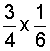Analysis: We will multiply two fractions.

Solution: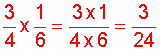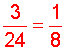Recall that the best way to simplify a fraction (reduce a fraction to lowest terms) is to find the greatest common factor of it's numerator and denominator; then divide both the numerator and the denominator by that same (nonzero) whole number.

Example 4: Multiply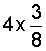Analysis: We will multiply a whole number by a fraction. Since 4 over 1 is the same as 4, we get.

Solution: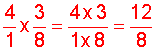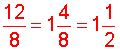In example 4, the GCF of 12 and 8 is 4. We could have simplified the result by cancelling (dividing out) a common factor of 4. This is shown below:Example 5: Multiply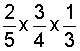Analysis: We will multiply three fractions.

Solution: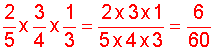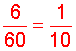Example 6: One batch of muffins contains 3/4 cups of raisins. How many cups of raisins are needed to make 6 batches of muffins?

Analysis: We will multiply a fraction by a whole number.

Solution: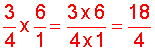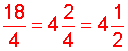We need 4 and 1/2 cups of raisins to make 5 batches of muffins.

Example 7: Multiply.Analysis: We will multiply an improper fraction by a proper fraction.

Solution:Summary: To multiply two or more fractions, multiply the numerators together and then multiply the denominators together. Simplify your result, if necessary.

### Exercises

Directions: Multiply the fractions in each exercise below. Be sure to simplify your result, if necessary. Click once in an ANSWER BOX and type in your answer; then click ENTER. After you click ENTER, a message will appear in the RESULTS BOX to indicate whether your answer is correct or incorrect. To start over, click CLEAR.

Note: To write the fraction three-fourths, enter 3/4 into the form. To write the mixed number four and two-thirds, enter 4, a space, and then 2/3 into the form.

 1.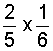ANSWER BOX:   RESULTS BOX:
 2.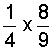ANSWER BOX:   RESULTS BOX:
 3.ANSWER BOX:   RESULTS BOX:
 4.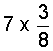ANSWER BOX:   RESULTS BOX:
 5. If it takes 2/5 meters of fabric to make a blouse, then how many meters will it take to make 9 blouses? ANSWER BOX:  m   RESULTS BOX:
 Lessons on Multiplying and Dividing Fractions and Mixed Numbers 1. Multiplying Fractions 2. Multiplying Fractions by Cancelling Common Factors 3. Multiplying Mixed Numbers 4. Reciprocals 5. Dividing Fractions 6. Dividing Mixed Numbers 6. Solving Word Problems 7. Practice Exercises 8. Challenge Exercises 9. Solutions